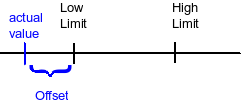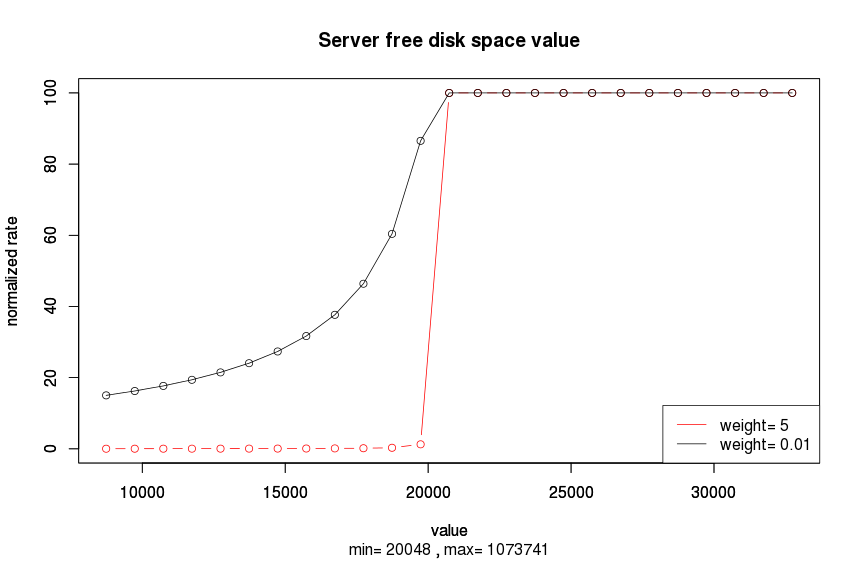# Job server rate computation - 7.2

Version
7.2
Language
English (United States)
Product
Talend Big Data
Talend Big Data Platform
Talend Data Fabric
Talend Data Integration
Talend Data Management Platform
Talend Data Services Platform
Talend ESB
Talend MDM Platform
Talend Real-Time Big Data Platform
Module
Content

## Job server rate computation

This document describes how execution servers in Talend Administration Center are assigned stars, in other words, how a server is said to be better than another for a Job execution.

The Job server is a probe running on the execution server. It will measure some features of the execution server such as the available memory, the available disk space, and so on. This information is sent to Talend Administration Center which then computes a rate value for this server.

A server has a set of features:

• the free disk space
• the free physical memory
• the free swap memory
• the idle CPU usage
• the nice CPU usage
• the total CPU usage
• the number of CPU

Some features are more important than the other. Therefore, you can weight these features to give more importance to some of them. This weight is set by the user in the monitoring_client.properties file. Let's call `weight{i}` the weight of the `i`th feature.

You can choose the range in which the feature is supposed to be good enough. This means you set some limits to be fulfilled by the feature of the server. For instance, a server is not a good server for the execution of the Job if it does not have 1Go of disk space. The lower limit on the disk would therefore be 1Go (to be set in the `monitoring_client.properties` file). Let's call `Min{i}` the lower limit defined on feature `i` and `Max{i}` its upper limit.

The server has an actual value for each feature. For instance, the server `i` has only 500 MB of free disk space. Let's call this value the actual value of the feature: `value{i}`.

The basic assumption is that the server is perfect as long as all of its features have actual values in the range defined by the limits. When some of its features have values outside the defined ranges, the server is not very good.

## How to compute the Job server rate

Let's define the offset of the feature as the difference between the limit and the actual value.Offset{i}= value{i} - Max{i}, when value{i} > Max{i} 0, when Min{i} < value{i} < Max{i} Min{i} - value{i}, when value{i} < Min{i}

The relative offset is given by the offset divided by the range:

``rel_offset{i} = offset{i} / [ Max{i} - Min{i} ]``

It's a positive value.

The rate of the server is computed as a weighted sum of all its relative offsets times a factor of -100:

``rate = -100 Σ weight{i} x rel_offset{i}``

At this stage, the rate is an unbounded negative number. In order to get a number between 0 and 100, the following formula is used:

``normalized rate = 100 / [ 1 - rate / scale ]``

where `scale = 2000`.

The scale is an arbitrary number that indicates the sensitivity to bad values. A normalized rate equal to 100 means that the server is good. A normalized rate lower than 100 means that the server is not so good. The lower the normalized rate is, the worst is the server. When all feature values are in the expected ranges, then the rate is 0 and the normalized rate is 100.

If the disk space is required to be between 1 GB and 2 GB and the actual disk space is 500 MB, then the relative offset is 1/2. The weight being 8, the normalized rate will be `100/ [ 1 + 400/2000 ] = 83.33`.

The below chart shows how the rate evolves according to the free disk space of the server and for two different weights (supposing that all other server features are inside the defined limits).The server rate updates each 90 seconds, which could be changed by setting the value of the parameter `notification.conf.checking.frequencyCheckJobServerState`.

Talend Administration Center lists rated servers in descending order, checks one by one from higher rate to lower rate to find out the best one, which should also have deployed Job.